# Square root (SQRT) in JavaScript

##### How to return the square root value in JavaScript?

Square Root

The square root of a number is a value that, when multiplied by itself, gives the number.

EXAMPLE: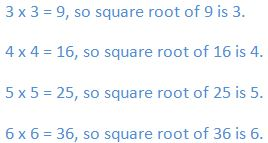In JavaScript, by using `SQRT` property & `sqrt()`Method we can return the square root value.

Difference between SQRT & sqrt

• Both `SQRT` & `sqrt()`  are used to find the value of square root in JavaScript.
• `SQRT` is a property, where as `sqrt()` is a method.
• `SQRT` property has only two parameters [`SQRT2`, `SQRT1_2`] in JavaScript. So we have to use only those two parameters to get the exact output. Incase if we enter the any input value, the output will show 'undefinied'.
• `sqrt()` method has no parameters, that means we can enter the input value manully in `sqrt()` method [Eg: `sqrt(36)`].
• `SQRT` property returns only the square root values of 2, 1/2. Where as `sqrt()` method returns square roots for all the input numbers, except the negative numbers.

Returning the square root values by using `SQRT` property.

EXAMPLE: Returning the Square root of 1/2 value (`SQRT1_2`).

```<p>Click the below button to return Square root.</p>
<input type="button" onclick="myNumber()" value="Click">
<p id="myId"></p>

<script>
function myNumber() {
document.getElementById("myId").innerHTML = Math.SQRT1_2;
}
</script>
```

In the above code snippet we have given `Id` as "`myId`"to the second `<p>` element in the HTML code. There is a function myNumber() in the`<script>`block which is connected to the onclick of the HTML button. We need to return the square root of 1/2 value, for that we are using`SQRT1_2`property. Onclick of the button "Click" in the HTML code fires the function myNumber() in the `<script>` block, at the same time `Math.SQRT1_2`property return the square root value of 1/2 as output.

OUTPUT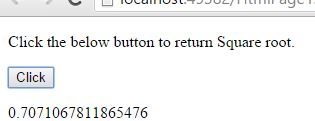### Returning the Square root of 2 value (`SQRT2`)

```<p>Click the below button to return Square root.</p>
<input type="button" onclick="myNumber()" value="Click">
<p id="myId"></p>

<script>
function myNumber() {
document.getElementById("myId").innerHTML = Math.SQRT2;
}
</script>
```

Square root of 2

Onclick of the button "Click" in the HTML code fires the function myNumber() in the `<script>` block, at the same time `Math.SQRT2`property return the square root value of 2 value as output.

OUTPUT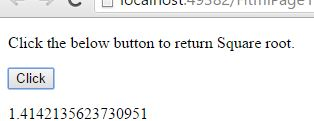Returning the values of square root by using `sqrt` property.

### Returning the square root value of 4 (`sqrt4`)

```<p>Click the below button to return Square root.</p>
<input type="button" onclick="myNumber()" value="Click">
<p id="myId"></p>

<script>
function myNumber() {
document.getElementById("myId").innerHTML = Math.sqrt(4);
}
</script>
```

Square root of 4

In the above code snippet we have given `Id` as "`myId`"to the second `<p>` element in the HTML code. There is a function myNumber() in the`<script>`block which is connected to the onclick of the HTML button. We need to return the square root of 4 value, for that we are using`sqrt(4)`property. Onclick of the button "Click" in the HTML code fires the function myNumber() in the `<script>` block, at the same time `Math.sqrt(4)`property return the square root value of 4 as output.

OUTPUT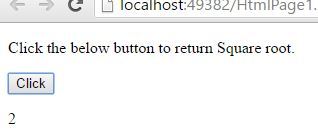### Returning the square root values for different numbers

```<p>Click the below button to return Square root.</p>
<input type="button" onclick="myNumber()" value="Click">
<p id="myId"></p>

<script>
function myNumber() {
var a = Math.sqrt(4);
var b = Math.sqrt(9);
var c = Math.sqrt(16);
var d = Math.sqrt(25);
var e = Math.sqrt(36);
var f = Math.sqrt(49);
var g = Math.sqrt(64);
var h = Math.sqrt(81);

var r = a + "<br>" + b + "<br>" + c + "<br>" + d + "<br>" + e + "<br>" + f + "<br>" + g + "<br>" + h;
document.getElementById("myId").innerHTML = r;
}
</script>
```

Square roots of different values

Onclick of the button "Click" in the HTML code fires the function myNumber() in the `<script>` block, at the same time `Math.sqrt()`property return the square root value of input numbers as output.

OUTPUT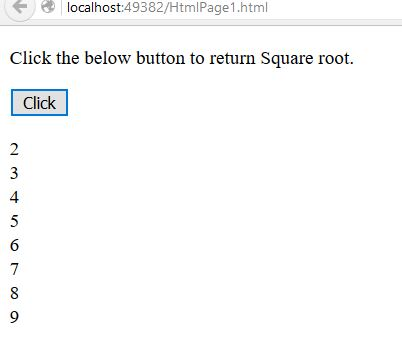NOTE: The `sqrt()` method returns output 'NaN' for the negative input numbers.

### Returning the square root value of '-36'

```<p>Click the below button to return Square root.</p>
<input type="button" onclick="myNumber()" value="Click">
<p id="myId"></p>

<script>
function myNumber() {
document.getElementById("myId").innerHTML = Math.sqrt(-36);
}
</script>
```

Square root of nageative value

In the above code snippet we have given negative value to the `sqrt()` method. The `sqrt() `method returns 'NaN' as output to the negative numbers.

OUTPUT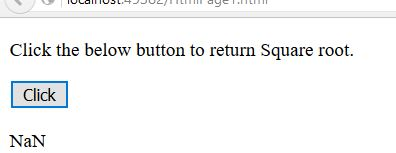What Euler's number, you will need to explain.
Views: 23286 | Post Order: 94